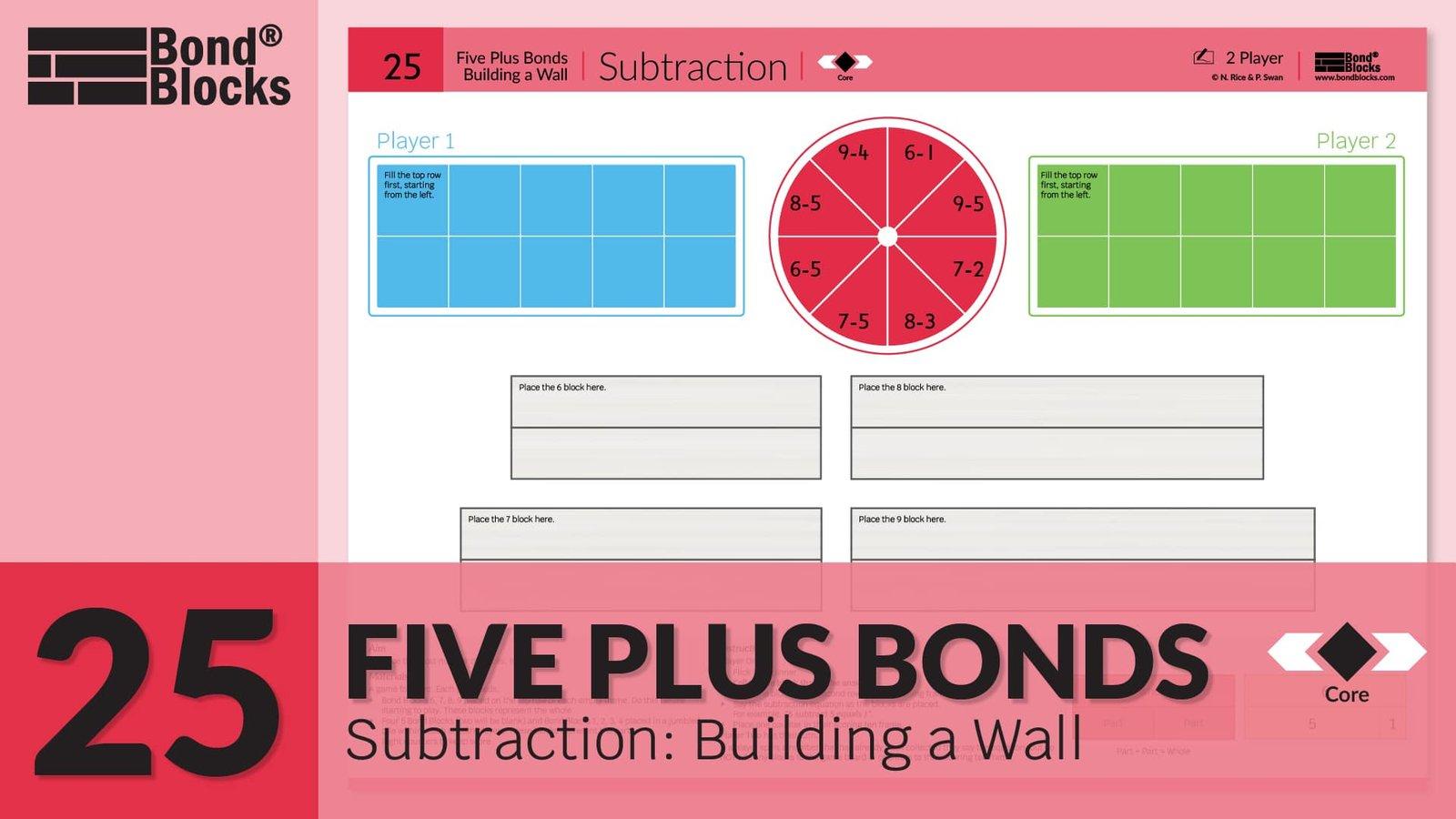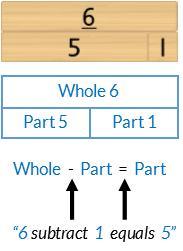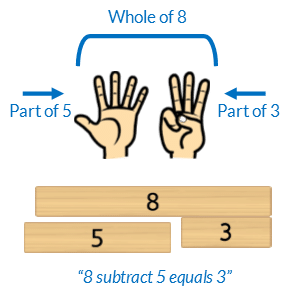## 25) Subtraction

Five Plus Bonds: Building a Wall### Mathematics

Develop fluency solving and verbalising subtraction equations for 6,7,8 and 9 partitioned as five plus bonds.

### Language

• subtraction as “subtract”
• “equals”
• part-part-whole## Differentiation

### A little easier

##### Represent five plus bonds with fingers

Instruct students who experience difficulty to make the amount using their fingers in a subitised way. One hand must be the part of 5.### Progression

In the next chapter of activities students develop different partitions for the whole of 10. The sequence of activities in the Bonds of 10 chapter is similar to past chapters: moving from identify bonds, to developing fluency, calculating, solving missing number equations and creating word stories. Go to

##### Activity 26

Bonds of 10: Bonds Building a Wall